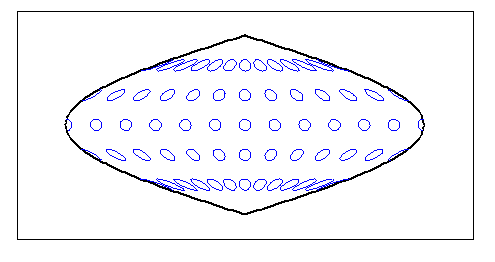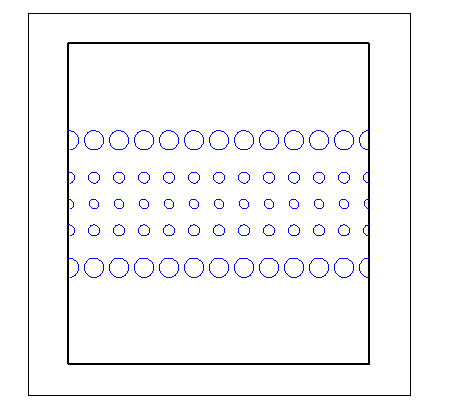# tissot

Project Tissot indicatrices on map axes

## Syntax

```h = tissot h = tissot(spec) h = tissot(spec,linestyle) h = tissot(linestyle) h = tissot(spec,PropertyName,PropertyValue,...) h = tissot(linestyle,PropertyName,PropertyValue,...) ```

## Description

`h = tissot` plots the default Tissot diagram, as described above, on the current map axes and returns handles for the displayed indicatrices.

`h = tissot(spec)` allows you to specify plotting parameters of the displayed Tissot diagram as described above.

`h = tissot(spec,linestyle)` and ```h = tissot(linestyle)``` where `linestyle` defines the style of the Tissot indicatrices, specified as a `linespec`.

`h = tissot(spec,PropertyName,PropertyValue,...)` and ``` h = tissot(linestyle,PropertyName,PropertyValue,...)``` allow the specification of any property and value recognized by the `line` function.

## Background

Tissot indicatrices are plotting symbols that are useful for understanding the various distortions of a given map projection. The indicatrices are circles of identical true radius on the Earth's surface. When plotted on a map projection, they indicate whether the projection has certain features. If the plotted indicatrices all enclose the same area, the projection is equal area (for example, a Sinusoidal projection would have this feature). If they all remain circular, then conformality is indicated (a Mercator projection has this property). Distortions in meridional or parallel distance are exhibited by flattened or stretched indicatrices. Many projections will show very even, circular indicatrices in some regions, often near the center, and wildly distorted indicatrices in others, such as near the edges. The Tissot diagram is therefore very useful in analyzing the appropriateness of a projection to a given purpose or region.

The general layout of the Tissot diagram is defined by the specification vector `spec`.

```spec = [Radius] spec = [Latint,Longint] spec = [Latint,Longint,Radius] spec = [Latint,Longint,Radius,Points]```

`Radius` is the small circle radius of each indicatrix circle. If entered, it should be in the same units as the map axes `Geoid`. The default radius is 1/10th the radius of the sphere.

`Latint` is the latitude interval between indicatrix circle centers. If entered it should be in the map axes `AngleUnits`. The default value is one circle every 30º of latitude (that is, 0º, +/-30º, etc.).

`Longint` is the longitude interval between indicatrix circle centers. If entered it should be in the map axes `AngleUnits`. The default value is one circle every 30º of latitude (that is, 0º, +/-30º, etc.).

`Points` is the number of plotting points per circle. The default is 100 points.

## Examples

```axesm sinusoid; framem tissot```The Sinusoidal projection is equal area.

`setm(gca,'MapProjection','Mercator')`The Mercator projection is conformal.